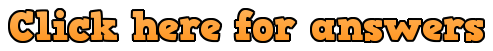Workout

Question 1

(a)  x = -5 and y = -2,   x = 1 and y = 4

(b)  x = -3 and y = -8,   x = 3 and y = -2

(c)  x = 1 and y = 1,   x = 3  and  y = 5

(d)  x = -4 and y = -3,   x = 1 and y = 12

(e)  x = -4 and y = 29,   x = -2  and  y = 7

(f)   x = -0.5 and y = -0.75,   x = 2  and  y = 8

Question 2

(a)  x = -5  and  y = 9,   x = 1 and  y = 3

(b)  x = 2  and  y = 5,    x = 5  and  y = 2

(c)  x = 2  and   y = 3,    x = 3  and  y = 2

(d)   x = -2  and  y = 0,    x = 3 and y = 10

(e)  x = -5  and  y = -2,   x = -2  and  y = -5

(f)  x = -6  and  y = 1,   x = 2  and  y = -3

(g)  x = 6 and y = 21,  x = 7  and  y = 31

(h)  x = -0.6 and y = 0.8,  x = 1 and y = 0

(i)  x = -2 and  y = 15,  x = 4  and  y = -15

(j)  x = -0.3333… and y = 0.3333…

(k)  x = 1 and y = 3,    x = 2.333.. and y = 0.333…

(l)  x = -9 and y = -2,   x = 23 and y = 6

Question 3

(a)  x = 3 and y = 1,   x = 6.333… and  y = -5.666..

(b)  x = -4.284 and y = -1.284,    x = 1.284  and  y = 4.284

(c)  x = -0.2915 and y = -0.2915,   x = 10.2915 and y = 10.2915

(d)   x = 1.14 and y = -2.72,   x = 2.19 and  y = -0.613

(e)  x = -4.46 and y = 8.42,  x = 1.46 and y = -3.42

(f)  x = -4.46 and y = -6.46,  x = 2.46 and y = 0.46

Apply

Question 1: (-3, 6) and (6, -3)

Question 2:  2 points of intersection and they are (-2, -10) and (2, -6)

Question 3:   The distance is √8 (or 2.8284) as the points are (-2, 2) and (0, 4)

Question 4:  (-3, 8) and (9/5, 176/25)

Question 5:  (2, 7) as only one solution, it is tangent

Question 6:  A and B are the points (2.25, -1.5) and (4, 2). Since the gradient of AB is 2 and the gradient of BC is -1/2, the lines are perpendicular. Therefore ABC is a right angled triangle.